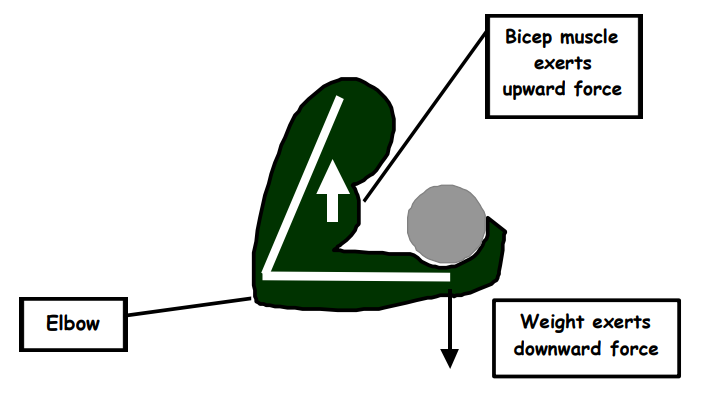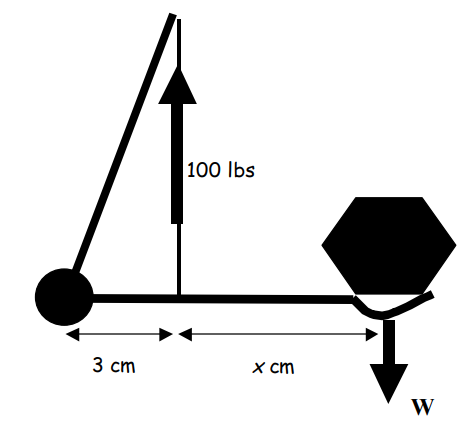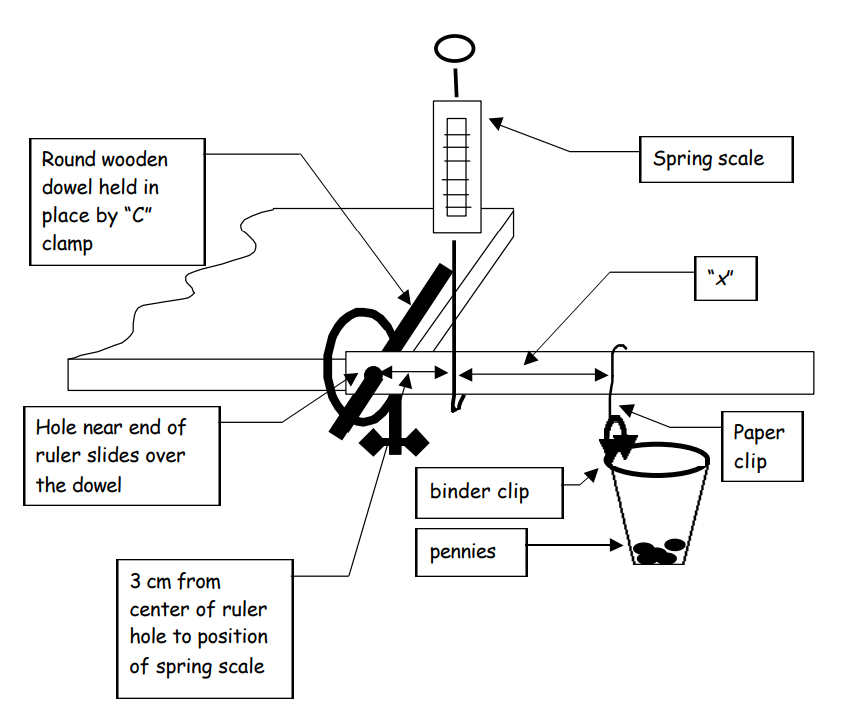Subject:
Mathematics
Science

Topics: AlgebraRational Equations and FunctionsForce
Concepts:

Mathematics:

· Rational expression

Science:

· Force

Knowledge and Skills:

Mathematics:

· Can solve equations involving rational expressions
· Can plot a rational function

Science:

· Can create a chart for the collection of experimental results
· Can present experimental results clearly in written form
· Can design an experiment which compares behavior or characteristics of classes of objects or subjects
· Knows that the amount of friction between two surfaces depends on the amount of pressure which pushes one surface against another
· Knows the units in which force is measured

Materials:

· One wooden or plastic ruler with a hole near one end
· A wooden dowel that just fits in the hole in the ruler
· One small C clamp
· One spring scale
· One heavy duty paper clip
· One binder clip
· A plastic cup (the type with a thickened rim)
· 200 pennies

Lesson:

Procedure: This project should be done by students individually or teams of two.

Distribute the handout and discuss it. Ensure that students understand the diagram. Review what they are being asked to do and ensure that they understand the assignment. Then have them begin.

Circulate as students work on the activity and assist as needed. Help them to determine just how they are going to investigate the questions asked, and help them work out how they are going to properly record their data.

Each student should prepare his or her own report on the procedure and results.

### Investigation: Leverage

This diagram shows how the biceps muscle is used to lift a weight:This diagram presents a model of the above situation:In this investigation you will make a model of the arm and investigate the relationships among some of the variables in the above situation.

1. Configure your equipment as shown in the diagram on the next page.

2. Position the “weight” so that x = 6 centimeters.

3. Determine how many pennies you must add in order for the spring scale to read exactly halfway on its scale, with the ruler is level.

4. Reposition the weight to a different value of x.

5. Again, determine how many pennies for the spring scale to be at the same position as above (exactly halfway).

6. Repeat for several different values of x. Create a table to keep track of the data (value of “x” and “number of pennies” in each instance).

7. Graph the data, with “x” as the independent variable and “number of pennies” as the dependent variable.

8. Describe the characteristics of the graph. What happens to “number of pennies” as “x” increases?

a) Which of the two functions below do you think is a better way to describe the relationship between the number of pennies, “n”, and the distance “x”?

linear function, n = ax + b

rational function, n = a/(b + x)

b) For the function you chose, find the values of “a” and “b” which give the closest match to the data you graphed.

c) What does your selected function predict for a value of “n” when x = 0?

d) Test this prediction.